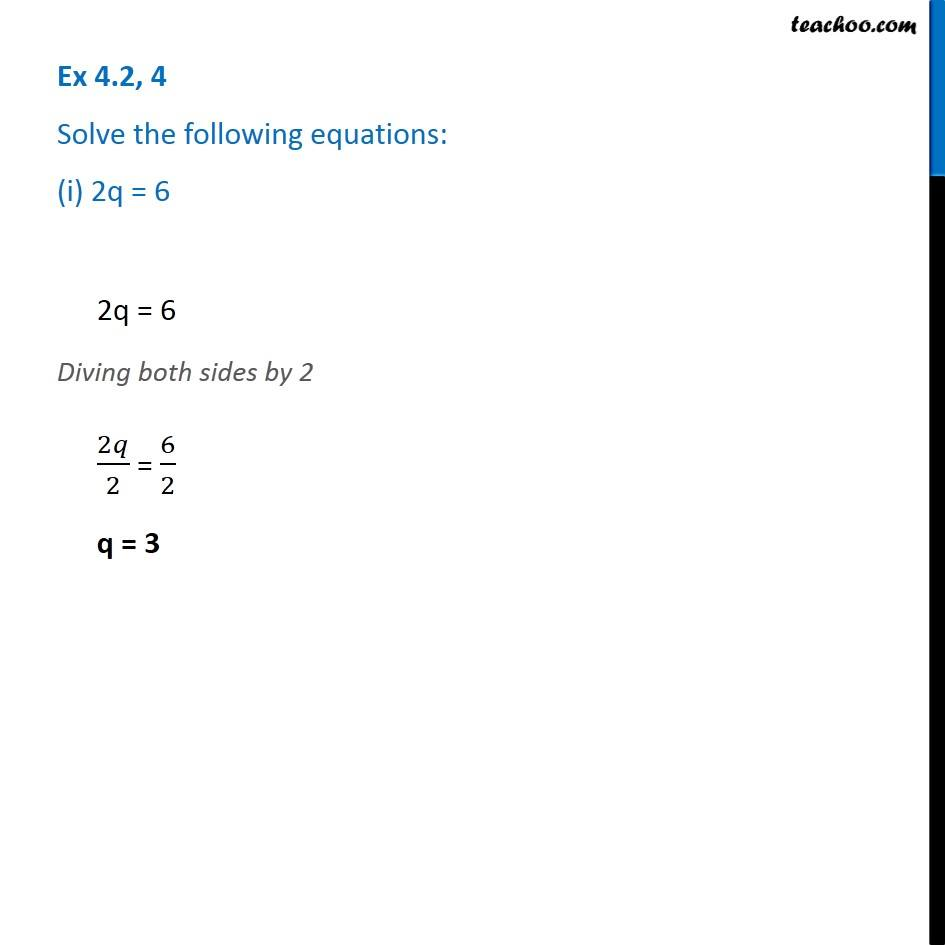1. Chapter 4 Class 7 Simple Equations
2. Serial order wise
3. Ex 4.2

Transcript

Ex 4.2, 4 Solve the following equations: (i) 2q = 62q = 6 Diving both sides by 2 2𝑞/2 = 6/2 q = 3